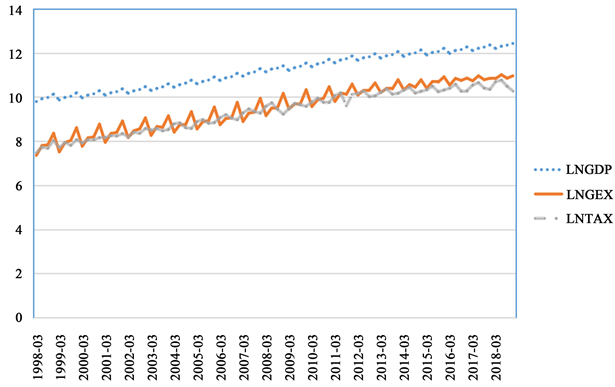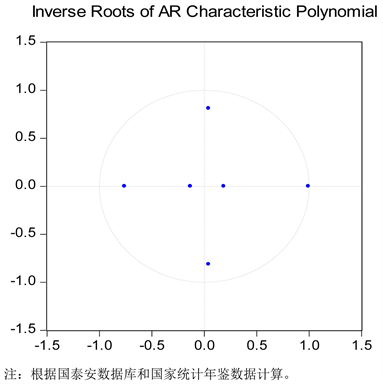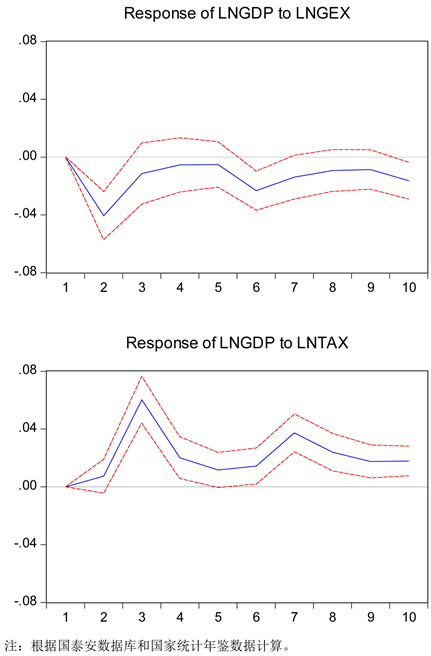﻿ 供给侧结构性改革视角下财政政策对经济增长的影响探求——基于VAR模型的脉冲响应分析

供给侧结构性改革视角下财政政策对经济增长的影响探求——基于VAR模型的脉冲响应分析 The Impact of Fiscal Policy on Economic Growth from the Perspective of Supply-Side Structural Reform—Impulse Response Analysis Based on VAR Model

Abstract: Fiscal policy is an important lever of economic growth, which cannot be ignored. Through the VAR model analysis of fiscal policy and economic growth, it is concluded that there is a significant statistical correlation between China’s fiscal policy and economic growth. But there is also a time lag, and the impact of fiscal policy tools on the fluctuation of economic growth is not the same. In light of the background of supply-side structural reform, we need to coordinate fiscal policies as a whole to ensure that they are both timely and prudent, streamline administration and delegate power, and work hard, so as to better serve economic development.

1. 引言

2. 文献综述

3. 财政政策促进经济增长

3.1. 财政政策概述

3.2. 经济增长现状

3.3. 财政政策作用于经济增长

4. 实证分析

4.1. 数据选取和统计性描述注：根据国泰安数据库和国家统计年鉴数据计算。

Figure 1. A logarithmic time-series of economic growth, fiscal expenditure, and taxationTable 1. ADF test results of each variableTable 2. Co-integration test results

4.3. VAR模型估计结果及分析

${Y}_{t}={A}_{0}+{A}_{1}{Y}_{t-p}+{A}_{2}{Y}_{t-p}+\cdots +{A}_{p}{Y}_{t-p}+{U}_{t}$Table 3. Selection table of optimal lag periods in VAR model

$\begin{array}{l}\text{LNGDP}=0.0697+0.6743*\text{LNGDP}\left(-1\right)+0.2869*\text{LNGDP}\left(-2\right)\\ \text{\hspace{0.17em}}\text{\hspace{0.17em}}\text{\hspace{0.17em}}\text{\hspace{0.17em}}\text{\hspace{0.17em}}\text{\hspace{0.17em}}\text{\hspace{0.17em}}\text{\hspace{0.17em}}\text{\hspace{0.17em}}\text{\hspace{0.17em}}\text{\hspace{0.17em}}\text{\hspace{0.17em}}\text{\hspace{0.17em}}\text{ }\text{\hspace{0.17em}}-0.2900*\text{LNGEX}\left(-1\right)-0.0809*\text{LNGEX}\left(-2\right)\\ \text{\hspace{0.17em}}\text{\hspace{0.17em}}\text{\hspace{0.17em}}\text{\hspace{0.17em}}\text{\hspace{0.17em}}\text{\hspace{0.17em}}\text{\hspace{0.17em}}\text{\hspace{0.17em}}\text{\hspace{0.17em}}\text{\hspace{0.17em}}\text{\hspace{0.17em}}\text{\hspace{0.17em}}\text{ }\text{\hspace{0.17em}}\text{\hspace{0.17em}}+0.0585*\text{LNTAX}\left(-1\right)+0.3639*\text{LNTAX}\left(-2\right)\end{array}$Figure 2. VAR model stability test

4.4. 格兰杰因果关系检验Table 4. Granger causality test results of VAR model

4.5. 脉冲响应函数Figure 3. Impulse response graph of LNGDP, LNGEX and LNTAX

4.6. 方差分解Table 5. Variance decomposition results of LNGDP

4.7. 结论与建议

 闫坤, 于树一. 当前宏观经济形势、供给侧结构性改革与财政政策[J]. 财经问题研究, 2017(2): 66-72.

 Smith, A. and Ebrary, I. (2013) An Inquiry into the Nature and Causes of the Wealth of Nations. Liberty Classics.

 Malthus, T.R. (1992) An Essay on the Principle of Population, or a View of Its Past and Present Effects on Human Happiness, with an Inquiry into Our Prospects Respecting the Future Removal or Mitigation of the Evils Which It Occasions (Rev. Ed.). Cambridge University Press, Cambridge.

 Keynes, J.M. (1935) The General Theory of Employment, Interest, and Money. Harcourt Brace and Co., Harcourt.

 刘伟, 蔡志洲. 经济增长新常态与供给侧结构性改革[J]. 求是学刊, 2016, 43(1), 56-65.

 徐寅. 新形势下深化财政政策在供给侧结构性改革中作用的研究[J]. 经济研究参考, 2017(52): 43-49.

 陶宗卫. 试论财政政策对于经济发展的作用[J]. 现代国企研究, 2018, 130(4): 109.

 徐蓓蓓. 人口老龄化作用下的财政政策经济增长效应研究[D]: [硕士学位论文]. 济南: 山东师范大学, 2015.

 李小平. 论财政政策对经济增长的促进效应[J]. 海南广播电视大学学报, 2008, 9(3): 73-77.

 马兰, 陈凯. 浅析财政政策对我国经济增长的贡献[J]. 现代经济信息, 2016(9): 4

 张峁, 王青, 乔东艳. 财政政策对经济增长和收入分配的长期影响效应分析[J]. 经济与管理, 2010, 24(2): 22-25.

 王春元. 我国政府财政支出结构与经济增长关系实证分析[J]. 财经研究, 2009, 35(6): 120-130.

Top